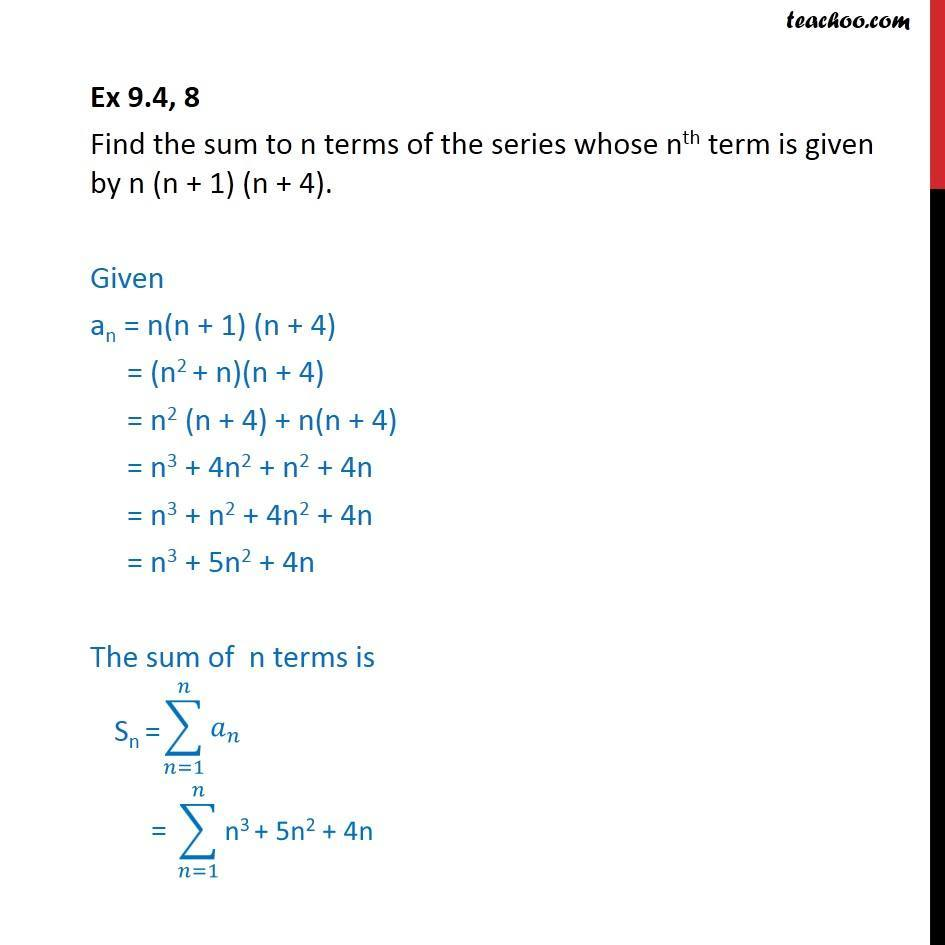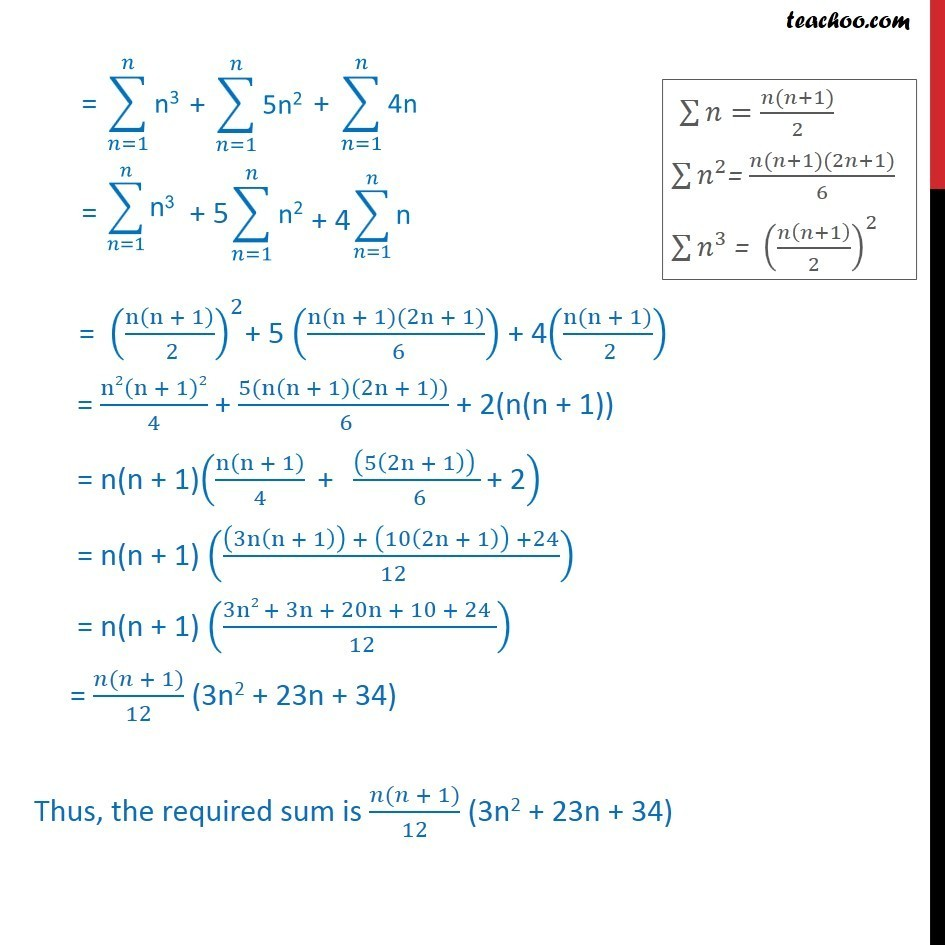Finding sum from nth number

Chapter 8 Class 11 Sequences and Series
Concept wiseLearn in your speed, with individual attention - Teachoo Maths 1-on-1 Class

### Transcript

Question 8 Find the sum to n terms of the series whose nth term is given by n (n + 1) (n + 4). Given an = n(n + 1) (n + 4) = (n2 + n)(n + 4) = n2 (n + 4) + n(n + 4) = n3 + 4n2 + n2 + 4n = n3 + n2 + 4n2 + 4n = n3 + 5n2 + 4n The sum of n terms is = (n(n + 1)/( 2))^2+ 5 ((n(n + 1)(2n + 1))/( 6)) + 4((n(n + 1))/( 2)) = n2(n + 1)2/4 + (5(n(n + 1)(2n + 1)))/( 6) + 2(n(n + 1)) = n(n + 1)(n(n + 1)/4 " + " ((5(2n + 1)) )/( 6) "+ 2" ) = n(n + 1) (((3n(n + 1)) + (10(2n + 1)) +24)/( 12)) = n(n + 1) ((3n2 + 3n + 20n + 10 + 24 )/( 12)) = (𝑛(𝑛 + 1))/12 (3n2 + 23n + 34) Thus, the required sum is (𝑛(𝑛 + 1))/12 (3n2 + 23n + 34)